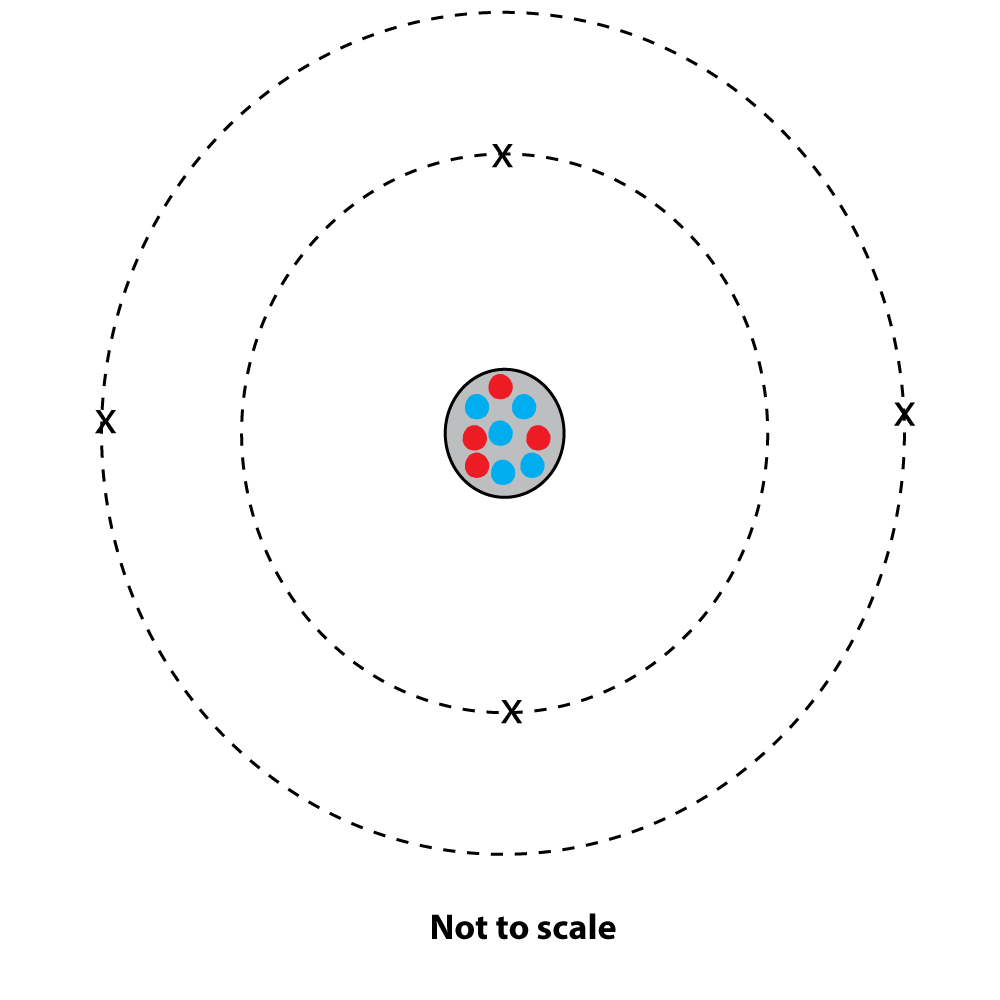µ GCSE | Atomic Structure

10 quick questions - for GCSE and iGCSE

10 minutes maximum! (can you do it in 5?)

1. Which particles are in the centre of an atom?

• A. protons and electrons.
• B. neutrons and electrons.
• C. neutrons and protons.
• D. protons, neutrons and electrons.

2. What is the name of the centre part of an atom, and what orbits around this?

 Centre part In orbit A nucleus protons B nucleole electrons C nucleus electrons D nucleole protons

Q3-6. Which of these particles....

3. ..has a negative charge.

4. ..has a mass of 1 atomic unit and a positive charge.

5. ..has a neutral charge.

Here is a diagram of an atom of Beryllium.

It has the symbol:6. What does the top number represent?

• A. The number of protons.
• B. The number of protons plus electrons.
• C. The number of neutrons.
• D. The total mass of the nucleus.

7. What does the bottom number represent?

• A. The number of protons.
• B. The number of protons plus electrons.
• C. The number of neutrons.
• D. The total mass of the nucleus.

8-10: Strontium isotopes

8. The symbol for the radioactive isotope strontium 90 is shown here. How many protons (p) and neutrons (n) does it have?

• A. 90 p, 90 n
• B. 38 p, 90 n
• C. 52 p, 38 n
• D. 38 p, 52 n9. Two different isotopes will have...

• A. ...the same number of protons but a different number of neutrons.
• B. ...the same number of neutrons but a different number of protons.
• C. ...the same number of protons but a different number of electrons.
• D. ...the same number of electrons but a different number of protons.

10. Question 8 descibes an isotope of strontium. Which of these describes the nucleus of a different isotope of strontium?

• A. 90 p, 91 n
• B. 39 p, 52 n
• C. 38 p, 53 n
• D. 53 p, 38 n